Question

# Consider the system of Figure(4) The reactances of all components in p.u, are indicated in Table (2)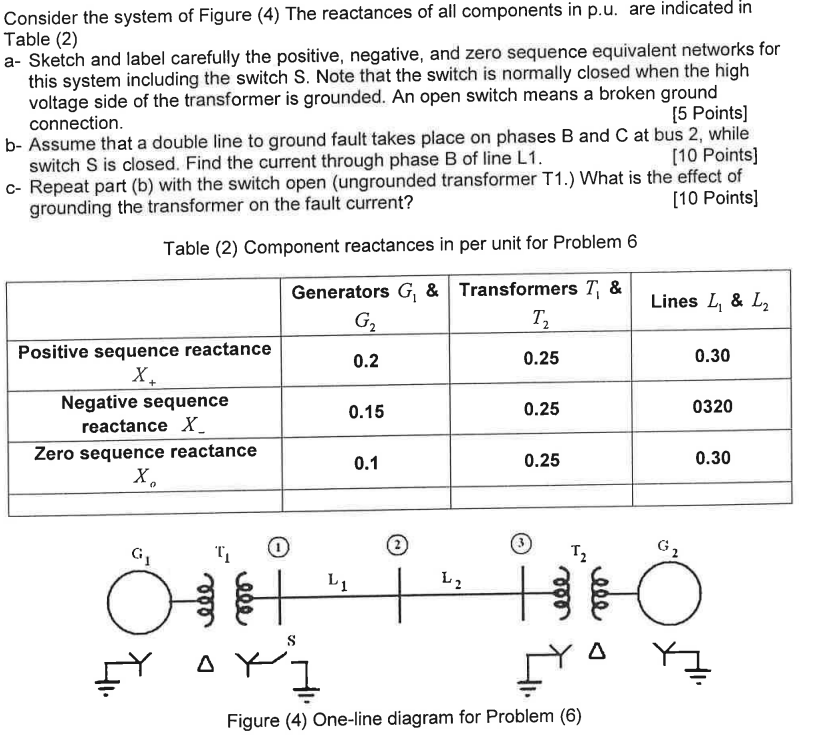We need at least 9 more requests to produce the answer.

1 / 10 have requested this problem solution

The more requests, the faster the answer.

All students who have requested the answer will be notified once they are available.

#### Earn Coins

Coins can be redeemed for fabulous gifts.

Similar Homework Help Questions
• ### Consider the system of Figure(4) The reactances of all components in p.u, are indicated in Table (2)Consider the system of Figure (4) The reactances of all components in p.u. are indicated in Table (2)  a- Sketch and label carefully the positive, negative, and zero sequence equivalent networks for this system including the switch S. Note that the switch is normally closed when the high voltage side of the transformer is grounded. An open switch means a broken ground connection. 15 Points)  b. Assume that a double line to ground fault takes place on phases B and...

• ### Consider the system of Figure(4) The reactances of all components in p.u, are indicated in Table (2)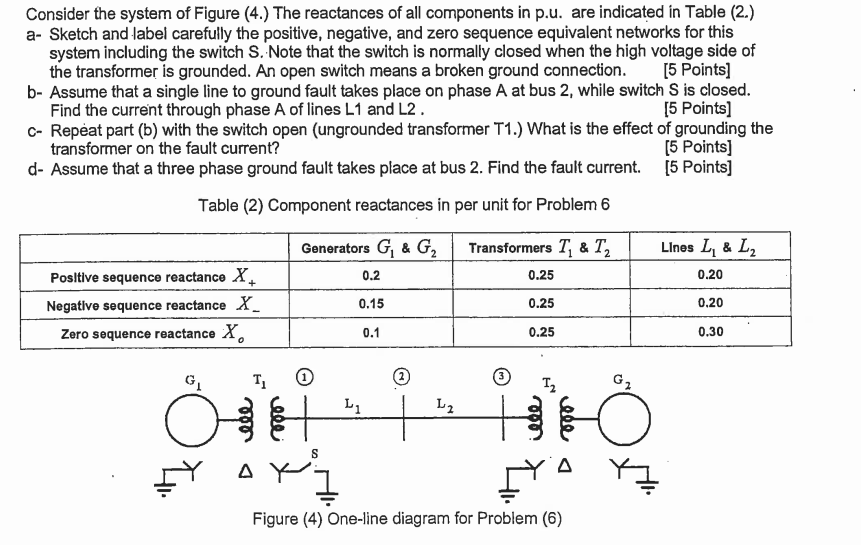• ### Consider the system of Figure. The reactances of all components in p.u, are indicated in Table (2)• ### Consider the system shown in the single-line diagram of Figure 3. All reactances are shown in...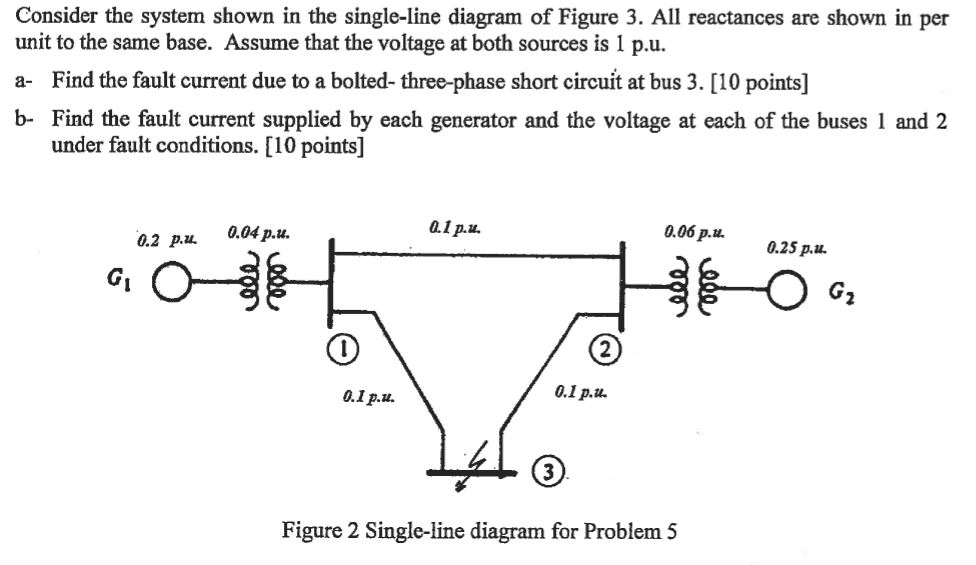Consider the system shown in the single-line diagram of Figure 3. All reactances are shown in per unit to the same base. Assume that the voltage at both sources is 1 p.u. a- Find the fault current due to a bolted- three-phase short circuit at bus 3. [10 points] b- Find the fault current supplied by each generator and the voltage at each of the buses 1 and 2 under fault conditions. [10 points] 0.1 p.u 0.06 ?.u. 0.25 p.11....

• ### Consider the system shown in the single-line diagram of Figure 3. All reactances are shown in...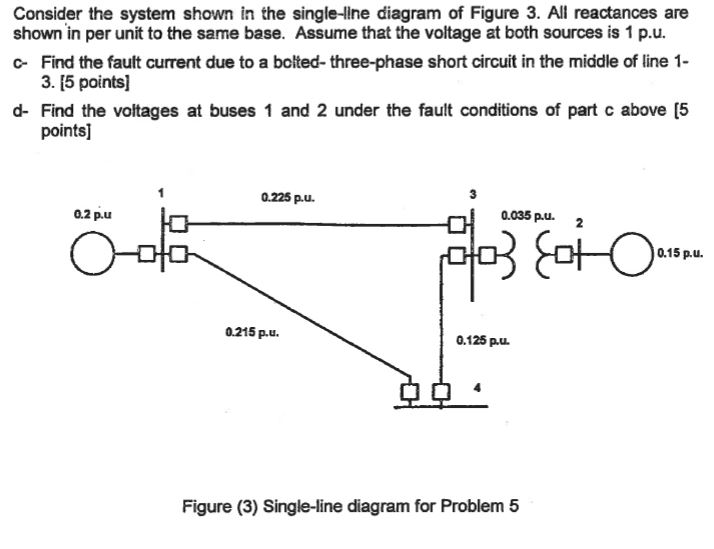Consider the system shown in the single-line diagram of Figure 3. All reactances are shown in per unit to the same base. Assume that the voltage at both sources is 1 p.u. c- Find the fault current due to a bolted-three-phase short circuit in the middle of line 1- 3. [5 points d- Find the voltages at buses 1 and 2 under the fault conditions of part c above [5 points] 0.225 pu. 0.035 pu. 0.2p.u ? 0.15 p.u. 0.215...

• ### Consider the system Shown in the single-line diagram of Figure 4. The required sequence reactances in per unit to the same base are as follows.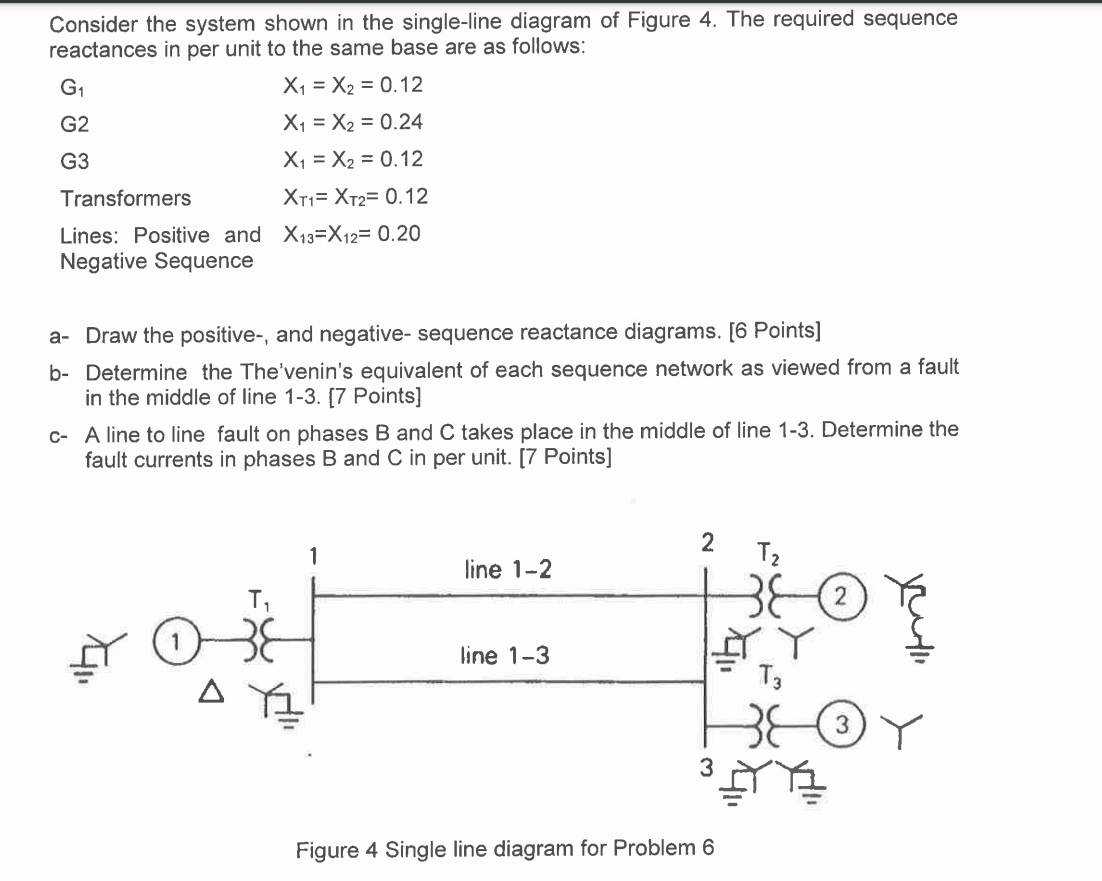Consider the system shown in the single-line diagram of Figure 4. The required sequence reactances in per unit to the same base are as follows: G1 X1 = X2 = 0.12  G2 X1 = X2 = 0.24  G3 X1 = X2 = 0.12  Transformers X11=X12= 0.12  Lines: Positive and X13=X12= 0.20 Negative Sequence  a. Draw the positive-, and negative- sequence reactance diagrams. [6 Points]  b- Determine the The'venin's equivalent of each sequence network as viewed from a fault in the...

• ### Consider the system Shown in the single-line diagram of Figure 4. The required sequence reactances in per unit to the same base are as follows.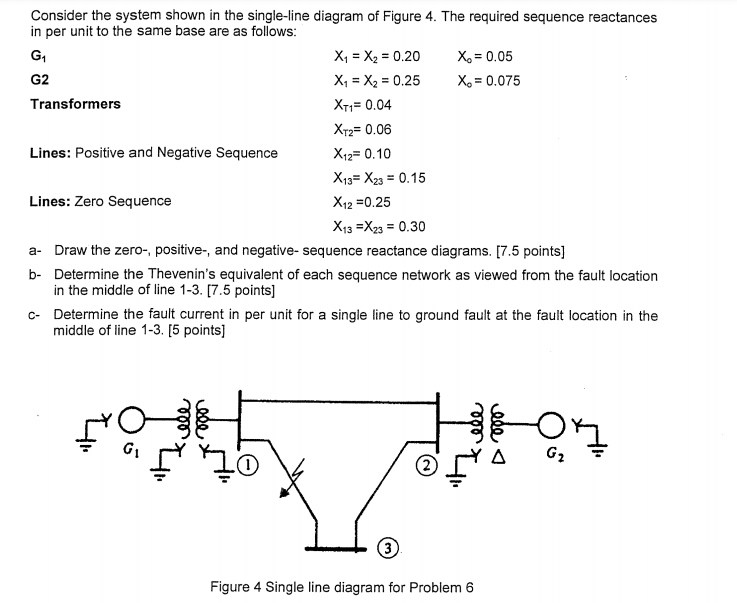• ### Consider the system Shown in the single-line diagram of Figure 4. The required sequence reactances in per unit to the same base are as follows.• ### Consider the system Shown in the single-line diagram of Figure (2). All reactance are shown in per unit to the same base. Assume that the voltage at both sources is 1 p.u.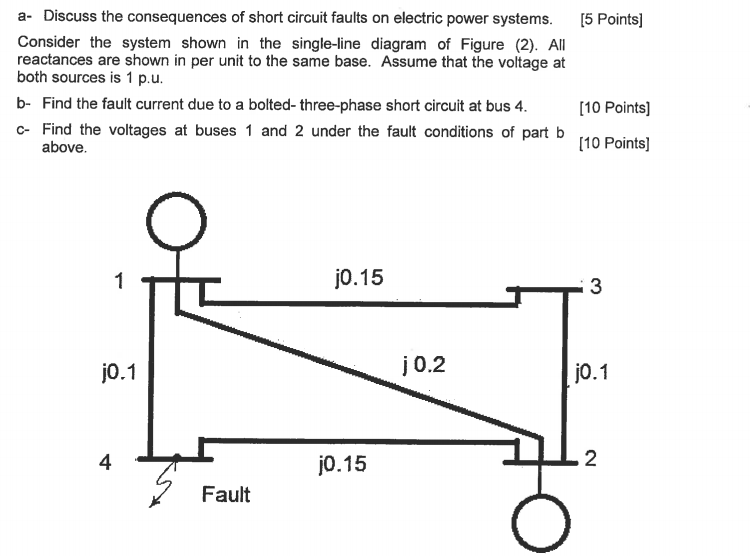• ### Consider the communication system shown in the accompany figure, with all components properly matched. If Pt 10 W and...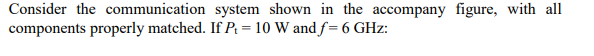Consider the communication system shown in the accompany figure, with all components properly matched. If Pt 10 W and f 6 GHz: (a) What is the power density at the receiving antenna (assuming proper alignment of antennas)? (b) What is the received power? (c) If Tsys 1,000 K and the receiver bandwidth is 20 MHz, what is the signal-to-noise ratio in decibels? SVS Gi-20 dB Gr 23 dB 20 km rec Rx Tx Consider the communication system shown in the...